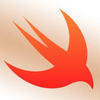## Welcome to the Treehouse Community

The Treehouse Community is a meeting place for developers, designers, and programmers of all backgrounds and skill levels to get support. Collaborate here on code errors or bugs that you need feedback on, or asking for an extra set of eyes on your latest project. Join thousands of Treehouse students and alumni in the community today. (Note: Only Treehouse students can comment or ask questions, but non-students are welcome to browse our conversations.)

### Looking to learn something new?

Treehouse offers a seven day free trial for new students. Get access to thousands of hours of content and a supportive community. Start your free trial today.

# how do i do this code

Using these values, we want to compute the product and print out the product in a formatted string.

Step 1: Declare a constant named product and assign the result of multiplying firstValue and secondValue together. (To multiply two values, a and b, we write a * b).

Step 2: Using string interpolation, create a string literal that describes the operation we just performed. For example, given the following values for firstValue, secondValue and product respectively: 2, 4, 8. The string should read: "The product of 2 times 4 is 8". Assign this string to a constant named output.

types.swift
```// Enter your code below
let firstValue = 2

let secondValue = 4

let product = "firstValue * secondValue"

let output = "2 * 4 \(product)"
```

i know you dont use the numbers at the bottom, it was a last resort hahaHi James

```let firstValue = 2
let secondValue = 4

//For this part you have it correct you do not need quotation marks around it though
let product = firstValue * secondValue

//You need to use string interpolation here exactly as the challenge states
let output = "The product of \(firstValue) times \(secondValue) is \(product)"
```